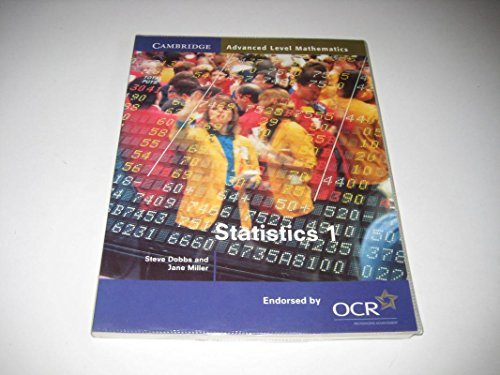Free Shipping on all orders in Australia
Over 7 million books in stock
Proud to be B-Corp# Statistics 1 for OCR by Steve Dobbs

Condition - Very Good
\$18.99
Only 2 left

## Summary

This book is part of the Cambridge Advanced Level Mathematics series, written specifically for the OCR modular mathematics syllabus. Each book or half-book corresponds exactly to one module. Statistics 1 has chapters on data representation, probability, expectation and variance, and the binomial distribution. It also introduces correlation and regression.

## Statistics 1 for OCR Summary

### Statistics 1 for OCR by Steve Dobbs

This book is part of the Cambridge Advanced Level Mathematics series, written specifically for the OCR modular mathematics syllabus. Each book or half-book corresponds exactly to one module. Statistics 1 has chapters on data representation, probability, expectation and variance, and the binomial distribution. It also introduces correlation and regression. Each chapter starts with a short list of learning objectives, and mathematical ideas are explained carefully and clearly, with many worked examples. There are plenty of exercises throughout, along with revision exercises and mock exam papers - all written by experienced examiners.

Introduction; 1. Representation of data; 2. Measures of Location; 3. Measures of spread; 4. Probability; 5. Permutations and combinations; 6. Random variables; 7. The binomial and geometric distributions; 8. Expectation and variance of a random variable; 9. Correlation; 10. Regression; Revision exercises; Mock examination papers; Answers; Index

GOR001468538
9780521786034
0521786037
Statistics 1 for OCR by Steve Dobbs
Used - Very Good
Paperback
Cambridge University Press
2000-06-15
222
N/A
Book picture is for illustrative purposes only, actual binding, cover or edition may vary.
This is a used book - there is no escaping the fact it has been read by someone else and it will show signs of wear and previous use. Overall we expect it to be in very good condition, but if you are not entirely satisfied please get in touch with us